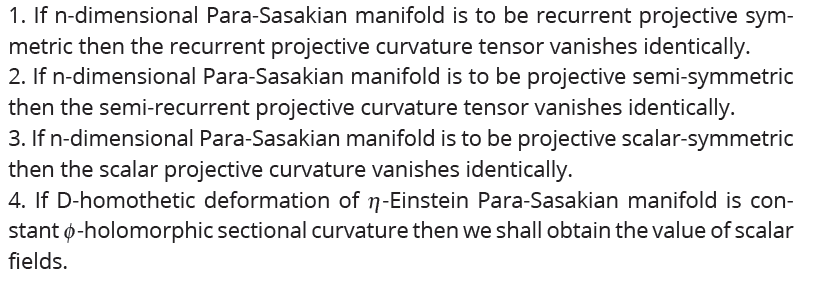• P-ISSN 0974-6846 E-ISSN 0974-5645# Indian Journal of Science and Technology

## Article• VIEWS 827
• PDF 284

Indian Journal of Science and Technology

Year: 2020, Volume: 13, Issue: 13, Pages: 1435-1439

Review Article

## D-homothetic deformation of an h-Einstein Para-Sasakian manifold

Received Date:08 April 2020, Accepted Date:23 April 2020, Published Date:25 May 2020

## Abstract

Objectives: The main purpose of this paper is to study the theory of Dhomothetic deformation of an h-Einstein Para-Sasakian manifold. Methods: A deformation technique is employed to solve the resulting problem. We provide its application in the general theory of relativity. Findings: Section 2 deals with recurrent and symmetric Para-Sasakian Manifolds. In section 3 we have defined and studied projectively symmetric Para-Sasakian manifold. The notion of ϕ -holomorphic sectional curvature in an h-Einstein Para-Sasakian manifold has been delineated in the section 4. In this very section, we shall study the relevant commutation formulae which give rise to the required curvature tensor. The obtained results were compared with the previous works(1--3) in this field and were found to be in good agreement. Novelty/Conclusion: The study concludes the following results:

1. If n-dimensional Para-Sasakian manifold is to be recurrent projective symmetric then the recurrent projective curvature tensor vanishes identically.
2. If n-dimensional Para-Sasakian manifold is to be projective semi-symmetric then the semi-recurrent projective curvature tensor vanishes identically.
3. If n-dimensional Para-Sasakian manifold is to be projective scalar-symmetric then the scalar projective curvature vanishes identically.
4. If D-homothetic deformation of h-Einstein Para-Sasakian manifold is constant ϕ -holomorphic sectional curvature then we shall obtain the value of scalar fields.

Keywords: ϕ -holomorphic sectional curvature; constant curvature; Para-Sasakian manifold; recurrent projective symmetric; D-homothetic deformation; h-Einstein manifold

## References

1. De K. D-Homothetic deformation of LP-Sasakian manifolds. Novi Sad Journal of Mathematics. 2015;45(2):113–123. doi: 10.30755/nsjom.2014.033
2. Dimri RC, Chauhan TS, Chauhan IS, Srivastava VK. A note on Einstein manifold in P-Sasakian manifold. International transactions in applied sciences. 2010;2(1):161–164.
3. Shaikh AA, Baishya KK, Eyasmin S. On D-Homothetic deformation of trans-Sasakian structure. Demonstratio Mathematica. 2008;XLI(1):171–188.
4. Chauhan I, Singh; Chauhan TS, Singh R, Kumar; Rizwan M. A note on D-Conformal para killing vector field in a P-Sasakian manifold. Journal of Xidian University. 2020;14:326–329.
5. Chauhan TS, Chauhan IS, Kumar. Rajendra: On recurrent and birecurrent Para-Sasakian manifolds. Acta Ciencia Indica. 2003;(1) 145–148.
6. Matsumoto M. T, NS., eds. On Riemannian spaces with recurrent projective curvature. (Vol. 19, pp. 11-18) 1968.Article Metrics